# Swift Algorithm Club: Graphs with Adjacency List

Learn how to implement directed and undirected graphs in Swift using adjacency lists. By Vincent Ngo.

Leave a rating/review
Save for later
Share

## Swift Algorithm Club: Graphs with Adjacency List

20 mins

Every month, Kelvin Lau, Chris Pilcher and I feature a data structure or algorithm on this site. If you want to learn more about algorithms and data structures, follow along with us!

In this tutorial, you’ll learn the basics of graph theory, then create an adjacency list in Swift 3.

Note: New to the Swift Algorithm Club? Check out our getting started post first.

## Getting Started

A Graph is a useful data structure to capture relationships between objects, made up of a set of vertices paired with a set of edges.

In the graph below, the vertices represents the circles, and the edges are the lines between them. A vertex connects to other vertices by edges.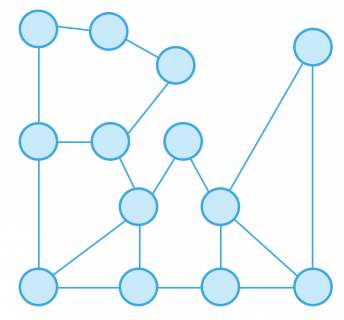Graphs can come in a variety of shapes and sizes.

### Weighted Graph

Take an airline for example. Imagine a network that shows varying routes for flights. Let the vertices represent the cities and the edges represent a possible route from city to city. Now you can associate a weight to every edge. From this network you can decipher the cheapest flights from San Francisco to Singapore.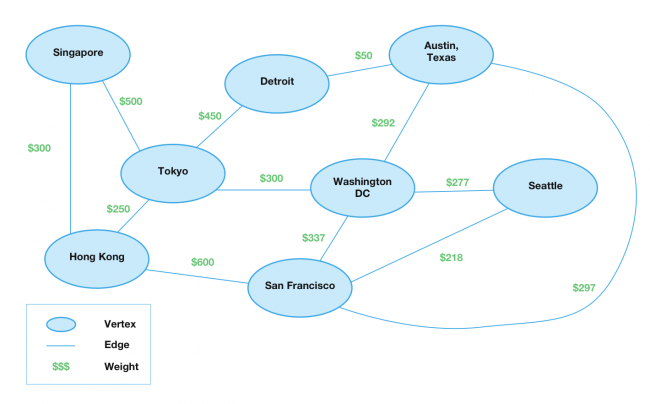The graph above represents a weighted graph where each edge has a numerical value.

### Directed and Undirected Graphs

A graph could also have direction. The first graph is a directed graph, where edges have direction. Imagine a flight from Toyko to Detroit, but no flight from Tokyo to Washington D.C.

A directed graph can also be bidirectional, where two vertices have two edges going back and forth. For example a flight from Singapore to Hong Kong, has a flight back as well.

The latter graph is an undirected graph, where there is no direction. In a way an undirected graph is just a directed graph that is bidirectional.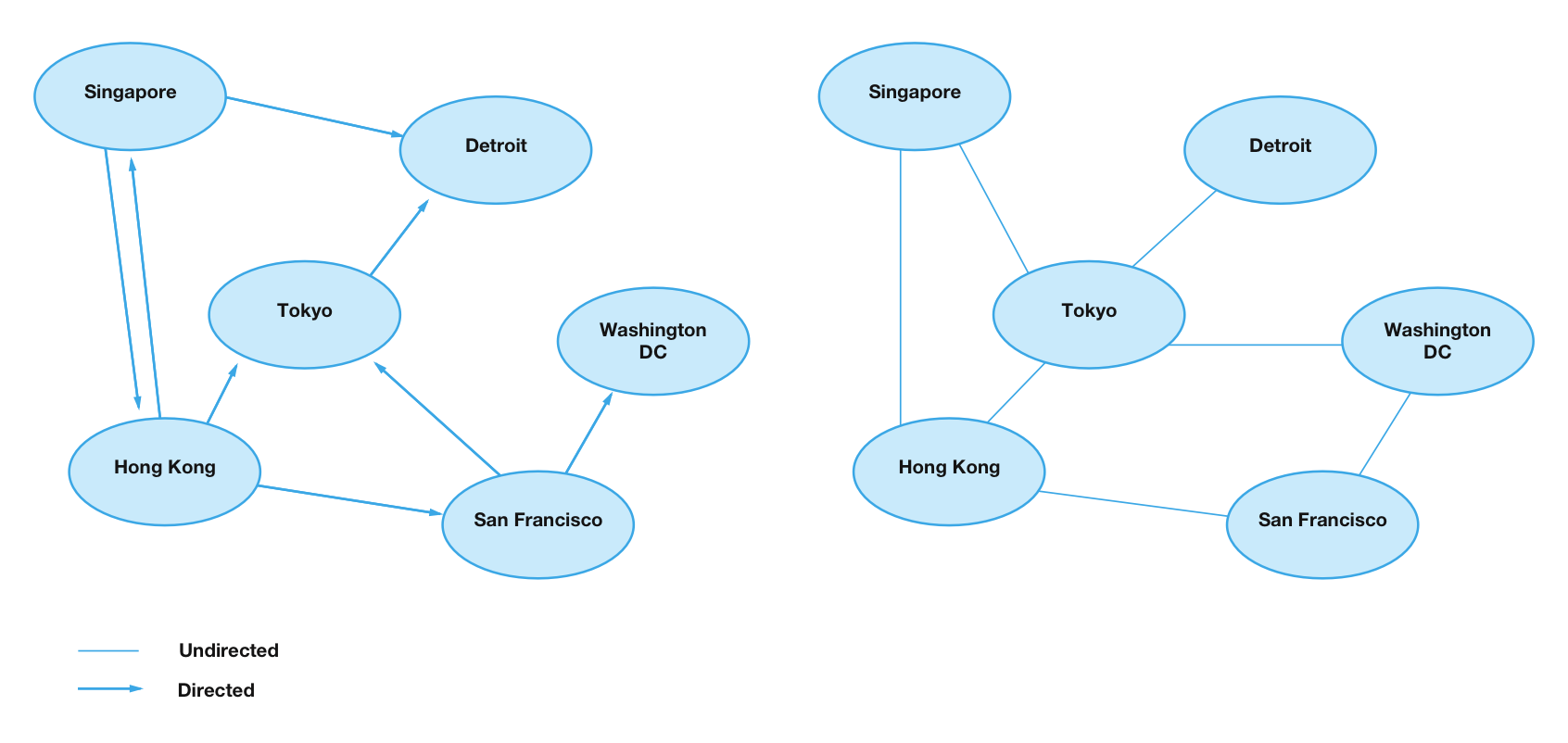You may have come across trees or linked-list data structures. They are a more simplified version of graphs.

## Representing a Graph

The two common ways to represent a graph is through an adjacency matrix or adjacency list. To get started with graphs, you will learn to create an adjacency list.

The basic idea of an adjacency list is you store every single vertex. Each vertex will hold an adjacency list. This describes the outgoing edges.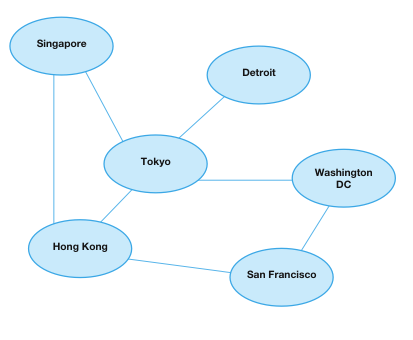The adjacency list below describes the flight network graph above.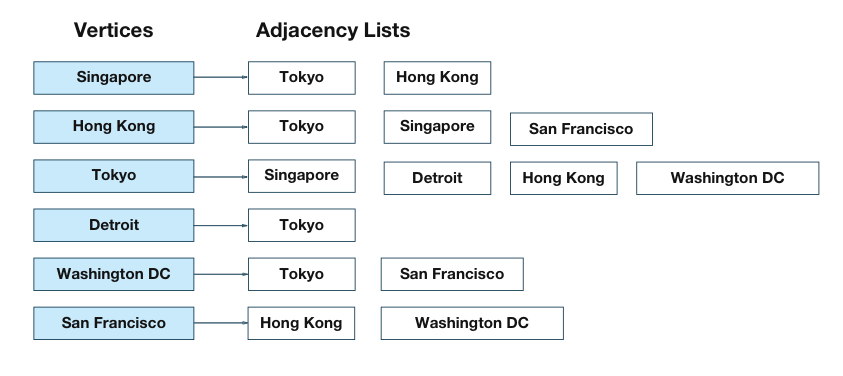As you can see the Singapore vertex has two edges associated with it. There are outgoing flights from Singapore to Toyko or Hong Kong.

### Approach

You can develop the adjacency list in many different ways. A few popular approaches are:

• Storing an array of arrays. The outer array represents vertices, providing an index. The inner array contains edges.
• Storing an array of linked-lists. With this approach, each index in the array represents a vertex. Each value in the array stores a linked-list. This is ideal if you need fast insertion or deletion times.
• Storing a dictionary of arrays. This is the approach you’ll take in this tutorial. Each key in the dictionary is a vertex, and each value is the corresponding array of edges.

Start by creating a new Swift playground called Graphs. In the Project Navigator (View\Navigators\Show Project Navigator, or ⌘-1), create a new file called Vertex.swift under the Sources group.

### Vertices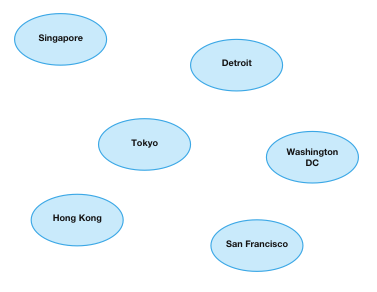The first thing that graphs need is a vertex. Add the following struct declaration in Vertex.swift:

```public struct Vertex<T: Hashable> {
var data: T
}
```

You’ve declared a struct named `Vertex`. The vertex holds a generic type called `data`. So now the vertex can represent pretty much any relationship, whether it’s airline flights, a person, or street addresses.

Next since you are storing a vertex as a custom key in a dictionary, it has to conform to the `Hashable` protocol. Add the following code below:

```extension Vertex: Hashable {
public var hashValue: Int { // 1
return "\(data)".hashValue
}

static public func ==(lhs: Vertex, rhs: Vertex) -> Bool { // 2
return lhs.data == rhs.data
}
}
```

Going over the code above:

1. For `Hashable` conformance you must provide a `hashValue` property.
2. `Hashable` inherits from the `Equatable` protocol. You must also add an equal-to operator for the custom type.

Now add the following code within the same file:

```extension Vertex: CustomStringConvertible {
public var description: String {
return "\(data)"
}
}
```

The `CustomStringConvertible` protocol allows you to define a custom output. You will use this to verify your adjacency list later.

### Edges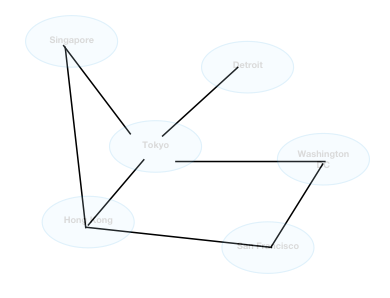Now to connect every vertex to another vertex, there needs to be an edge between them! Create a new file called Edge.swift under Sources group.

First add the following enum to Edge.swift

```public enum EdgeType {
case directed, undirected
}
```

The goal of the enum is to describe whether an edge between two vertices is a `directed` path, or `undirected` path.

Now add the following code after:

```public struct Edge<T: Hashable> {
public var source: Vertex<T> // 1
public var destination: Vertex<T>
public let weight: Double? // 2
}
```

Going over the properties in `Edge`: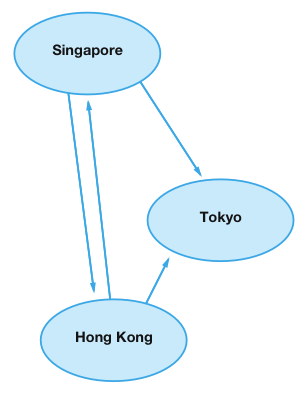Take this graph as an example. The flight between Singapore and Hong Kong has two edges between the pair of flights. This means the flight goes both ways. But, there is only one edge between Singapore and Tokyo. There are only flights that go from Singapore to Tokyo but not back.

1. There are many ways to represent an edge depending on your implementation. For this edge, it’s property includes two vertices, `source` and `destination`. The reason is because graphs can be directional. Two vertices are bidirectional, would need two edges between the pair of vertices.
2. An edge could also have a numerical weight. This is useful for future algorithms. You may want to find out how much it costs to fly from Hong Kong to Tokyo.

Like in the `Vertex` struct, add the following code in Edge.swift:

```extension Edge: Hashable {

public var hashValue: Int {
return "\(source)\(destination)\(weight)".hashValue
}

static public func ==(lhs: Edge<T>, rhs: Edge<T>) -> Bool {
return lhs.source == rhs.source &&
lhs.destination == rhs.destination &&
lhs.weight == rhs.weight
}
}
```

`Edge` conforms to the `hashable` protocol. For similar reasons to the `Vertex` struct.Vincent Ngo

Author